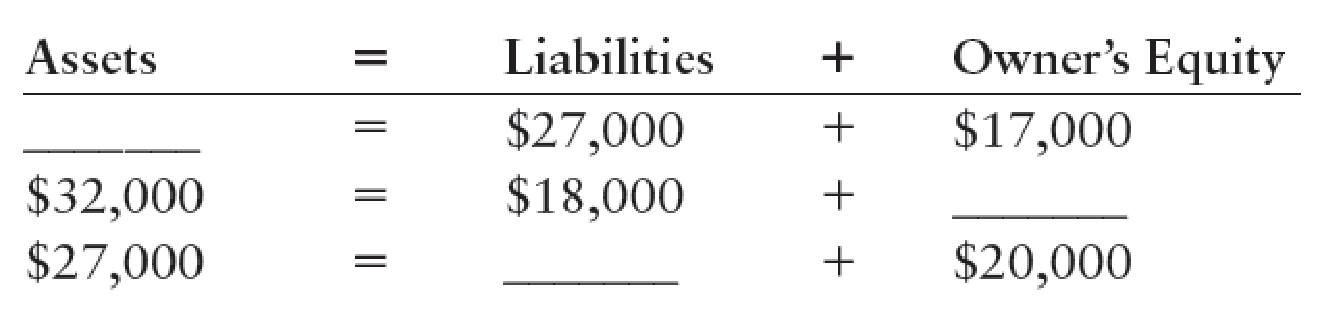Chapter 2, Problem 2SEA

Chapter
Section
Textbook Problem

THE ACCOUNTING EQUATION Using the accounting equation, compute the missing elements.To determine

Compute the missing elements by using the accounting equation.

Explanation

Accounting equation: Accounting equation is an accounting tool expressed in the form of equation, by creating a relation between resources or assets of a business and claims on the resources by the creditors, and the owners.

Calculate the missing elements:

 Assets = Liabilities + Owners' equity $44,000 (1) =$27,000 $17,000$32,000 = $18,000$14,000 (2) $27,000 =$7,000 (3) $20,000 Table (1) Working note: Calculate the value of assets: Assets=Liabilities+Owners'equity=$27,000

Still sussing out bartleby?

Check out a sample textbook solution.

See a sample solution

The Solution to Your Study Problems

Bartleby provides explanations to thousands of textbook problems written by our experts, many with advanced degrees!

Get Started